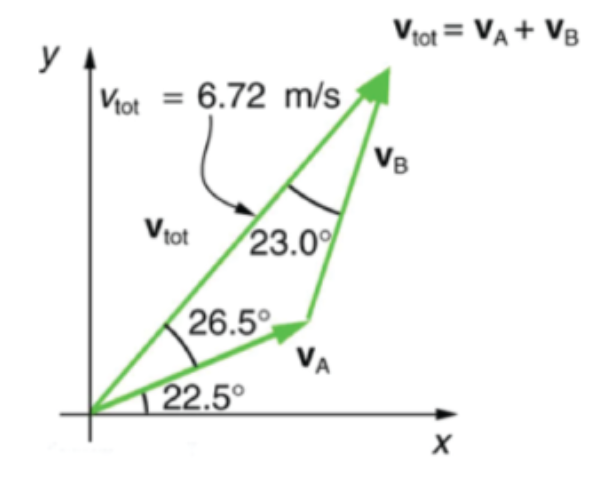# Problem: The figure depicts the sum of two velocitiesPart (a) Find the component, in meters per second, of vtot along an x’-axis rotated 30° counterclockwise relative to the x-axis in the figure.vtot x’=Part (b) Find the component, in meters per second, of vtot along a y’-axis rotated 30° counterclockwise relative to the y-axis in the figure

###### FREE Expert Solution

In this problem, we are required to rotate the coordinate system and resolve a vector into its components.

2D vector Components:

82% (361 ratings)###### Problem Details

The figure depicts the sum of two velocitiesPart (a) Find the component, in meters per second, of vtot along an x’-axis rotated 30° counterclockwise relative to the x-axis in the figure.

vtot x’=

Part (b) Find the component, in meters per second, of vtot along a y’-axis rotated 30° counterclockwise relative to the y-axis in the figure